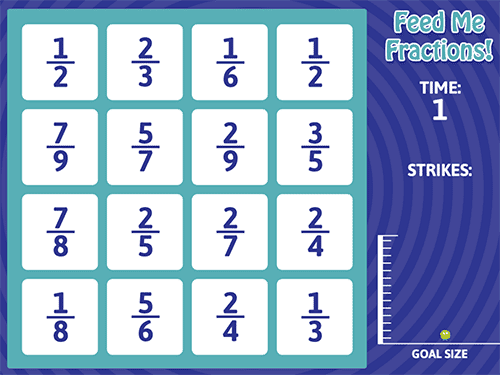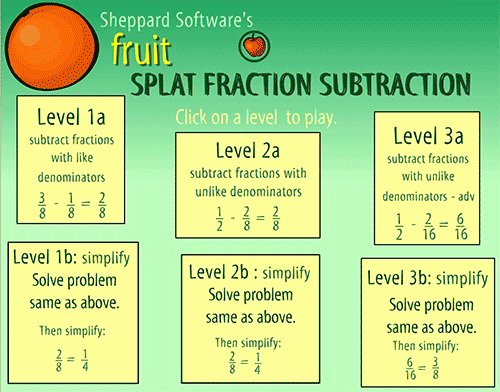Friday, 19 November 2021

Unit 4 - Adding and subtracting fractions

Hello, hello!
This unit on fractions is ending and I want to share with you some resources to practice how to add and subtract (with like and unlike denominators).Here's the explanation:How to add fractions - Maths is FunHow to substract fractions - Maths is fun
Exercises and games on adding fractions:Addition of fractions - Easy levelAddition of fractions - Hard levelFeed me fractions - Easy levelAdding fractions - Unlike denominators game
Exercises and games on subtracting fractions:Subtracting fractions - Hard levelSplat fraction subtraction game - Different levelsSubtracting exercise with unlike denominators
Games on both addition and subtraction of fractions:Adding and substracting fractions - Race car gameAdding and subtracting fractions game
I hope you find this useful!
Bye, bye, butterflies! 🦋